## Saturday, February 21, 2009

### How (not) to compute harmonic numbers

The nth harmonic number is the nth partial sum of the divergent harmonic series, Hn = 1 + 1/2 + 1/3 + ... + 1/n. The simplest way to compute this quantity is to add it directly the way it is written: 1, 1+1/2, 1+1/2+1/3, and so on. For n approximately greater than 10 or 100, this is algorithm is not a very good one. Why?

Firstly, in floating-point arithmetic, it is much better to use the asymptotic expansionwhich requires fewer terms the larger n is. Combining this with recurrence when n is too small gives a fast numerical algorithm for fixed-precision or arbitrary-precision computation of harmonic numbers (especially large ones). It allows one to determine, for example, that it takes exactly 15092688622113788323693563264538101449859497 terms until the partial sums of the harmonic series exceed 100. (This algorithm is implemented in mpmath, and most other comparable software.)

Secondly, the naive algorithm is especially bad for exact computation of harmonic numbers. This might not be obvious at a glance. We are just adding n numbers: what's to improve? The catch is that rational arithmetic has hidden costs: a single addition requires three multiplications and usually a GCD reduction. In contrast with integer or floating-point addition, rational addition gets very slow when the numerators and denominators get large. Computing harmonic numbers the naive way triggers worst-case behavior of rational arithmetic: the denominators grow like n! (if no GCD is performed, the denominator of Hn literally becomes n!).

It should be well known that computing n! as 1, 1·2, 1·2·3, ... is a poor method when n is large. A much better algorithm, assuming that multiplication is subquadratic, is to recursively split the products in half, i.e. compute (1·2·...·n/2) · ((n/2+1)·...·n) and so on. This algorithm has complexity O(log(n) M(n log(n)) compared to O(n2 log(n)) for the naive algorithm, where M(n) is the complexity of multiplying two n-bit integers. This approach is called binary splitting, and has many other applications.

The example of factorials directly suggests an analogous algorithm for computing harmonic numbers with reduced complexity: just recursively split the summation in half.

A few more variations are also possible. Instead of working with rational numbers, which require a GCD reduction after each addition, one can work with the unreduced numerators and denominators as integers, and form a rational number at the end. Another way to obtain a pure-integer algorithm for Hn = p/q is to compute the denominator q = n! and then use the formulafor the numerator.

Below, I have implemented five different algorithms for computing harmonic numbers based on the preceding remarks. The code works with either Python 2.6, using the Fraction type from the standard library, or with gmpy, by adding the following respective header code:

`# pythonfrom math import factorial as facfrom fractions import Fraction as mpqone = 1mpz = int# gmpyfrom gmpy import mpz, mpq, facone = mpz(1)`

Algorithm 1: directly add 1, 1/2, 1/3, ..., 1/n:
`def harmonic1(n):    return sum(mpq(1,k) for k in xrange(1,n+1))`

Algorithm 2: like algorithm 1, GCD reduction postponed to the end:
`def harmonic2(n):    p, q = mpz(1), mpz(1)    for k in xrange(2,n+1):        p, q = p*k+q, k*q    return mpq(p,q)`

Algorithm 3: compute denominator; compute numerator series:
`def harmonic3(n):    q = fac(n)    p = sum(q//k for k in xrange(1,n+1))    return mpq(p,q)`

Algorithm 4: binary splitting:
`def _harmonic4(a, b):    if b-a == 1:        return mpq(1,a)    m = (a+b)//2    return _harmonic4(a,m) + _harmonic4(m,b)def harmonic4(n):    return _harmonic4(1,n+1)`

Algorithm 5: binary splitting, GCD reduction postponed to the end:
`def _harmonic5(a, b):    if b-a == 1:        return one, mpz(a)    m = (a+b)//2    p, q = _harmonic5(a,m)    r, s = _harmonic5(m,b)    return p*s+q*r, q*sdef harmonic5(n):    return mpq(*_harmonic5(1,n+1))`

I did some benchmarking on my laptop (32-bit, 1.6 GHz Intel Celeron M), with n up to 104 for the Python version and 106 for the gmpy version. Here are the results (times in seconds):

`python:n         harmonic1  harmonic2  harmonic3  harmonic4  harmonic510         0.000159   0.000009   0.000010   0.000160   0.000025100        0.004060   0.000233   0.000234   0.002211   0.0003581000       0.889937   0.028822   0.029111   0.048106   0.02643410000    769.109402   3.813702   3.823027   2.710409   3.475280gmpy:n         harmonic1  harmonic2  harmonic3  harmonic4  harmonic510         0.000028   0.000010   0.000010   0.000033   0.000021100        0.000284   0.000097   0.000111   0.000365   0.0002261000       0.003543   0.001870   0.004986   0.004280   0.00265110000      0.103110   0.142100   0.588723   0.059249   0.052265100000     7.861546  17.002986  74.712460   1.115333   1.2008651000000  994.548226      -           -     27.927289  24.425421`

Algorithm 1 clearly does not do well asymptotically. For the largest n measured, it is 284 times slower than the fastest algorithm with Python arithmetic and 40 times slower with gmpy arithmetic. Interestingly, algorithms 2 and 3 both substantially improve on algorithm 1 with Python arithmetic at least up to n = 10000, but both are worse than algorithm 1 with gmpy. This is presumably because GMP uses a much better GCD algorithm that reduces the relative cost of rational arithmetic.

Algorithms 4 and 5 are the clear winners for large n, but it is hard to tell which is better. The timings seem to fluctuate a bit when repeated, so small differences in the table above are unreliable. I think algorithm 5 could be optimized a bit if implemented in another language, by accumulating temporary values in machine integers.

It is amusing to note that computing the 10000th harmonic number with the recursive algorithm and an optimized implementation of rational arithmetic is 14,700 times faster than the direct algorithm with a non-optimized implementation of rational arithmetic. Choosing a larger n (say, 105) gives an even more sensational ratio, of course, which I'm not patient enough to try. The moral is: Moore's law is not an excuse for doing it wrong.

Algorithm 1 is not all bad, of course. With memoization (or implemented as a generator), it is good enough for sequential generation of the numbers H1, H2, H3, ..., and this tends to be needed much more commonly than isolated large harmonic numbers. SymPy therefore uses the memoized version of algorithm 1.

The algorithms above can be adapted for the task of computing generalized harmonic numbers, i.e. numbers of the form 1 + 1/2k + 1/3k + ... + 1/nk for some integer k. Also worth noting is that algorithm 5 allows for computation of Stirling numbers of the first kind. Since |S(n+1,2)| = n! · Hn, algorithm 5 can be viewed as an efficient algorithm for simultaneous computation of S(n,2) and n!. Expressing Stirling numbers in terms of generalized harmonic numbers, this extends to an algorithm for S(n,k) for any (large) n and (small) k.

A final remark: binary splitting is not the fastest algorithm for computing factorials. The fastest known method is to decompose n! into a product of prime powers and perform balanced multiplication-exponentiation. Can this idea be applied to harmonic numbers and/or Stirling numbers? It's not obvious to me how it would be done. Are there any other, possibly even faster algorithms for harmonic numbers? I've looked around, but been unable to find anything on the subject.

## Thursday, February 19, 2009

### Python 3.1 twice as fast as 3.0

For large-integer arithmetic, that is. Mark Dickinson has beeen working on a patch that changes the internal representation of long integers from 15-bit to 30-bit digits, and there is an additional patch that adds a few algorithmic optimizations, written by Mark and Mario Pernici. Likely both patches will pass review and be included in Python 3.1. I asked Mark to run the mpmath unit tests to find out how big a difference the patches make, which he kindly did. Here are the results:
`    32-bit build:    unpatched (15-bit digits): 42.91 seconds    patched (30-bit digits): 40.57 seconds    patched+optimized: 30.15 seconds    64-bit build:    unpatched: 38.39 seconds    patched: 22.36 seconds    patched+optimized: 21.55 seconds    patched+optimized+bitlength: 20.20 seconds`

The last result includes the use of the new int.bit_length() method (which I had a small part in implementing) instead of the pure-Python version in mpmath.

It's not surprising that the patches make high-precision arithmetic much faster, but most of the mpmath tests actually work at low precision and depend mostly on general speed of the Python interpreter. There the speedup is perhaps of order 0-30%. For the tests working at very high precision, the improvement is a factor 4 or 5 with the 64-bit build. Then there are a number of tests in between. With some imagination, all unit tests together provide a plausible estimate of actual performance for a wide range of applications.

An excerpt of before/after results for some particular tests, comparing 64-bit unpatched and 64-bit patched+optimized+bitlength:
`    # Only tests 53-bit precision    double_compatibility      ok        1.3149240 s    double_compatibility      ok        1.1979949 s    # Logarithms up to 10,000 digits    log_hp                    ok        4.0845711 s    log_hp                    ok        0.7967579 s    # Large Bernoulli numbers    bernoulli                 ok        6.8625491 s    bernoulli                 ok        1.4261260 s    # Gamma function, high precision    gamma_huge_2              ok        2.4907949 s    gamma_huge_2              ok        0.5781031 s    # Numerical quadrature, 15 to 100 digit precision    basic_integrals           ok        3.0117619 s    basic_integrals           ok        1.8687689 s`

Mpmath does not yet run on Python 3.x; the benchmarking was made with a version of mpmath hacked slightly for the tests to pass. It would be nice to provide a 3.x compatible version of mpmath soon. The 2to3 tool fortunately handles almost all necessary patching; a handful of fairly simple additional changes need to be made. The most annoying problem is the lack of cmp (and particularly the cmp argument for sorted), which has no trivial workaround, but still should not be too hard to fix. In any case, it seems likely that the 30-bit patch will also be backported to Python 2.7, so most users should be able to take advantage of it.

It would be interesting to compare these benchmarks with the GMPY mode in mpmath. GMPY too provides a ~2x speedup for the unit tests. (This factor would be much larger if tests at even higher precision were included. At a million digits, GMPY is orders of magnitude faster than pure Python.) Originally GMPY only sped up the very high precision tests, and was otherwise slower than pure Python, probably due to Python's internal optimizations for int and long instances. This disadvantage was eliminated by implementing some helper functions for mpmath in C in GMPY. Mario Pernici has recently worked on further optimizations along the same lines, which should substantially improve low-precision performance.

## Tuesday, February 17, 2009

### Approximate prime counting

I'm continuing my quest for the special functions coverage in mpmath to match the coverage in Mathematica. Comparisons are not quite fair, because mpmath is (with a few exceptions) numerical-only, and it is missing some heavy-duty functions such as the Meijer G-function (and will for the foreseeable future). Many functions in mpmath are also implemented on smaller domains and less rigorously. On the other hand, Mathematica is more restricted in that it only supports machine-precision exponents. And most importantly, the Mathematica source code is very difficult to read for most people.

In any case, I'm trying to catch up with Mathematica 7, which added a whole slew of functions (not that I've caught up with Mathematica 6 or even 5). I did add a few functions in version 0.11 of mpmath as a consequence of seeing them in Mathematica 7 (as I recall right now, the Barnes G-function, hyperfactorial and generalized Stieltjes constants). See also the "Fun with zeta functions" post, which discussed the addition of the Riemann-Siegel functions in mpmath.

I just committed an implementation of the Riemann R function R(x) (also discovered in the "new in Mathematica 7" list), which is an analytic function that closely approximates the prime counting function π(x). The incredible accuracy of the Riemann R function approximation can be visualized by plotting it against the exact prime counting funtion (I added a naive implementation of π(x) as primepi, mainly to facilitate this kind of comparison -- SymPy contains a slightly more optimized primepi which could be used instead):
`>>> from mpmath import *>>> plot([primepi, riemannr], [0,100])`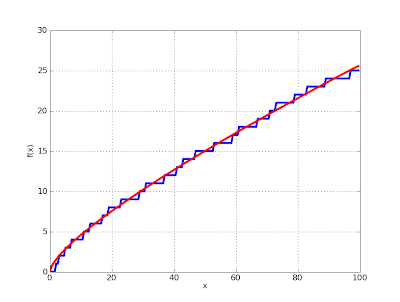The accuracy for small x is not a fluke, either:
`>>> plot([primepi, riemannr], [100000,105000])`The "classical" approximation based on the logarithmic integral is not nearly as good:
`>>> plot([primepi, lambda x: li(x) - li(2)], [100000,105000])`The largest exact value of the prime counting function ever computed was π(1023). The Riemann R function is quite easy to evaluate for x far larger than that, and the relative accuracy only improves:
`>>> mp.dps = 50>>> print riemannr(10**1000)4.3448325764011974554109300516481778421781159070188e+996`

It is quite likely that no one will ever compute the exact integer that is π(101000), but the value shown above is correct to every indicated digit. In fact, R(101000) can be shown using simple estimates to be accurate to about 500 digits.

For the fun of it, I implemented a version of the prime counting function that quickly returns a strict bounding interval for π(x), using an inequality by Lowell Schoenfeld:This gives for example:
`>>> mp.dps = 15>>> print primepi2(10**9)[50823160.0, 50875310.0]`

Comparing the Riemann R function and the exact prime counting function with the lower and upper bounds of the Schoenfeld inequality:
`>>> pi1 = lambda x: primepi2(x).a>>> pi2 = lambda x: primepi2(x).b>>> plot([primepi, riemannr, pi1, pi2], [10000,12000])`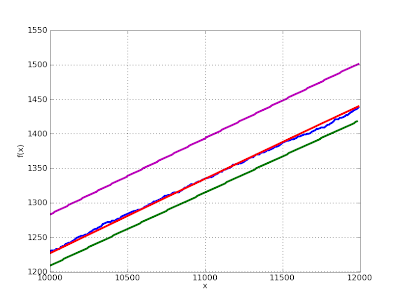Returning to the example x = 101000, the bounds obtained are:
`>>> mp.dps = 1000>>> v = primepi2(10**1000)>>> print v.a4344832576401197455410930051648177842178115907018753520641877406789202895629742141091652903064607718165071467289250162191865437538175693583349276176983162304817200288422839930447222611946361773708166613649511865077141255564428528876728476273763948755679190328683931767101119581057633512778001576529635483244120948895852940270929343735968992442702495339548061518980068329967842108151105502256086735702708401375364414161249392284219849396842747077740262581952863984834051417932416644877138920521584769223323611487747350418739878648537734638421782508735661222272164076496151428324862762772509621406939237565543669854423809507199075669125082789947534727993213855318461906160646707017635974631675017156972857845295824952837298472639509394842782539243804497572144916796756352176142163031921644787290530029234804577579707557749789078595077525265053645288083485194663875002648919670801486005071068990914291696975675686030935121708700243252203694578706535251850297014373844901850360974589491752273505884338.0>>> print v.b4344832576401197455410930051648177842178115907018753520641877406789202895629742141091652903064607718165071467289250162191865437538175693583349276176983162304817200288422839930447222611946361773708166613649511865077141255564428528876728476273763948755679190328683931767101119581057633512778001576529635483244120948895852940270929343735968992442702495339548061518980068329967842108151105502256086735702708401375364414161249392284219849396842747077740262581952863984834051417932416644877138920521603092613295597181269170104433360015451521965223364742414453272450659125521224590219319931601764655406966919866611954863306151010025269888571358859446324550862963908106206882475628658096023618959670013112886111645738414968101094745943938600966894156016440525536572293671667091549374215308487923845943703593204613524632469949425914711588697691782286908198073032336714125348156636382686152398749263039694035970181319549445806688213828419222097880911972939154885202053608277109394003350202108994842650400209.0>>> nprint((v.delta)/v.a)4.21728e-495>>> nprint((riemannr(10**1000)-v.a)/v.a)2.10863e-495`

and so it turns out that "accurate to about 500 digits" can be replaced by "accurate to at least 494 digits".

Actually, this is not quite true. Schoenfeld's inequality depends on the truth of the Riemann hypothesis. Thus, it is conceivable that primepi2 might return an erroneous interval. If you find an output from primepi2 that you can prove to be wrong, this must mean either that 1) you've found a bug in mpmath, or 2) you've disproved the Riemann hypothesis. (One of these options is more likely than the other, and reports should therefore be submitted to the mpmath issue tracker rather than Annals of Mathematics.)

On a final note, I've also added the function zetazero for computing the nth nontrivial zero of the Riemann zeta function:
`>>> mp.dps = 50>>> print zetazero(1)(0.5 + 14.134725141734693790457251983562470270784257115699j)>>> print zetazero(2)(0.5 + 21.022039638771554992628479593896902777334340524903j)>>> print zetazero(10)(0.5 + 49.773832477672302181916784678563724057723178299677j)>>> nprint(zeta(zetazero(10)))(2.14411e-50 - 4.15422e-50j)`

It just uses a table to determine correct initial values for the rootfinder, so zetazero currently only works up to n = 100. In fact, it works up to n = 100,000 on an internet-enabled computer; zetazero will automatically try to download the table of 100,000 zeros from Andrew Odlyzko's website if called with n > 100. Or it can load a custom url/file if specified by the user. This is one reason why I love Python: downloading a data file, parsing it and converting it to an appropriate internal representation in virtually a single line of code, easily done in the middle of some algorithmic code.

More information about the Riemann R function can be found in "The encoding of the prime distribution by the zeta zeros", part of Matthew R. Watkins' amazing website. The moral of that document is that the error between the exact prime counting and the Riemann R function can be represented by a kind of "Fourier series" involving a summation over the zeros of the Riemann zeta function. I have not gotten to looking at that yet.

## Wednesday, February 4, 2009

### Galerkin's method in SymPy

I'm currently taking a PDE course, and for this reason I am trying to come terms with the Galerkin method. The Galerkin method is conceptually simple: one chooses a basis (for example polynomials up to degree q, or piecewise linear functions) and assumes that the solution can be approximated as a linear combination of the basis functions. One then chooses the coefficients so as to make the error orthogonal to all basis functions; this amounts to computing a sequence of integrals (the inner products determining orthogonality) and then solving a linear system of equations.

Doing the calculations by hand can get very messy. Fortunately, it is straightforward to implement the Galerkin method e.g. for an ODE IVP in SymPy and have the ugly parts done automatically. The following function implements the global Galerkin method on an interval [x0, x1], using polynomials up to degree q as the basis. It is assumed that the 0th degree basis function is fixed to the initial value.

`from sympy import *sum = __builtins__.sumdef galerkin(ode, x, x0, x1, u0, q):    basis = [x**k for k in range(q+1)]    # Coefficients for the basis monomials    xi = [Symbol("xi_%i" % k) for k in range(q+1)]    # Solution function ansatz    u = u0 + sum(xi[k]*basis[k] for k in range(1,q+1))    # Form system of linear equations    equations = [integrate(ode(u)*basis[k], (x, x0, x1)) \        for k in range(1,q+1)]    coeffs = solve(equations, xi[1:])    return u.subs(coeffs)`

In general, the integrals can get very complex, if not unsolvable, and one must fall back to numerical methods. But for a simple problem, SymPy can calculate the integrals and the symbolic solution provides insight. Solving the standard problem u' - u = 0 (with solution u(x) = exp(x)):

`x = Symbol('x')ode = lambda u: u.diff(x) - ufor q in range(1,6):    pprint(galerkin(ode, x, 0, 1, 1, q))`

This outputs:
`1 + 3*x              2    8*x   10*x1 + --- + -----     11     11               2       3    30*x   45*x    35*x1 + ---- + ----- + -----     29     116     116                  2        3        4    1704*x   882*x    224*x    126*x1 + ------ + ------ + ------ + ------     1709     1709     1709     1709                     2         3         4        5    31745*x   15820*x    5460*x    1050*x    462*x1 + ------- + -------- + ------- + ------- + ------     31739     31739      31739     31739    31739`

One can see that the coefficients rapidly approach those of Maclaurin polynomials for exp. The fast global convergence is also readily apparent upon visualization:

`from mpmath import plotu1 = Lambda(x, galerkin(ode, x, 0, 1, 1, 1))u2 = Lambda(x, galerkin(ode, x, 0, 1, 1, 2))u3 = Lambda(x, galerkin(ode, x, 0, 1, 1, 3))plot([exp, u1, u2, u3], [0, 2])`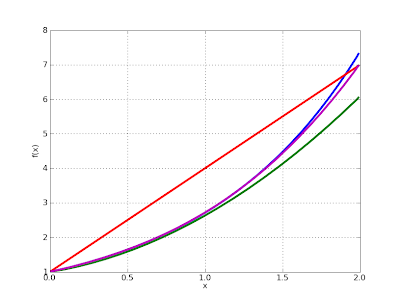The blue curve is exp(x). Note that the plotted interval is larger than the solution interval, so already the q = 3 solution (purple) is essentially accurate to within graphical tolerance. With moderately high degree, one gets a very accurate solution:

`>>> galerkin(ode, x, 0, 1, 1, 10).subs(x,'7/10').evalf()2.01375270747023>>> exp('7/10').evalf()2.01375270747048`

The Galerkin method might prove useful as an alternative algorithm for high-precision ODE solving in mpmath. Mpmath has no problem with the nonlinear integrations, nor with solving the linear system in case it gets ill-conditioned at higher degree.

I'll perhaps post some more code on this topic in the future, e.g. for solving the ODE with trigonometric and piecewise polynomial basis functions.

### Fun with zeta functions

In mpmath-trunk there is now an implementation of the Riemann-Siegel Z function as well as the related Riemann-Siegel theta function. There is also a function for computing Gram points.

A picture is worth a thousand words. The Z function is a kind of real-valued version of the Riemann zeta function on the critical strip (compare to this previous plot):

`>>> from mpmath import *>>> plot(siegelz, [0,80])`The implementation is entirely generic, so it works in the complex numbers:

`>>> cplot(siegelz, points=50000)`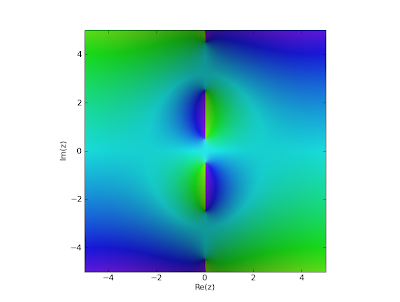`>>> cplot(siegelz, [-25,25], [-25,25], points=50000)`Gram points are useful for locating zeros of the zeta/Z functions. Huge Gram points themselves are easily located:

`>>> mp.dps = 50>>> print grampoint(10**10)3293531632.7283354545611526800803306343201329980271`

Unfortunately, evaluation of the Riemann zeta or Riemann-Siegel Z functions is not feasible for such large inputs with the present zeta function implementation in mpmath. Lowering expectations a bit, one can still compute some fairly large zeros:

`>>> g1 = grampoint(1000)>>> g2 = grampoint(1001)>>> r = findroot(siegelz, [g1, g2], solver='illinois')>>> print r1421.8505671870486539107068075509847506037846486061>>> print g1 < r < g2True>>> nprint(siegelz(r))-1.30567e-51`

(I chose the Illinois solver here because it combines bracketing with the fast convergence of the secant method.)

A plot of the initial couple of Gram points:

`>>> mp.dps = 15>>> plot(lambda x: grampoint(floor(x)), [0, 30])`The following visualization of Gram points is perhaps more illustrative. It is usually the case that the Gram points and sign changes (i.e. zeros) of Z alternate with each other:

`>>> gs = [grampoint(n) for n in range(25)]>>> def marker(x):...     for g in gs:...         if abs(g-x) < 0.2:...             return 1...     return 0...>>> plot([siegelz, marker], [0,50])`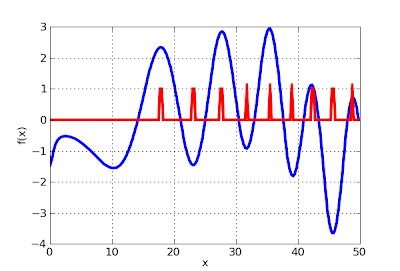Put another way, it is usually the case that (-1)n Z(gn) is positive (this is Gram's law):

`>>> (-1)**10 * siegelz(grampoint(10)) > 0True>>> (-1)**11 * siegelz(grampoint(11)) > 0True>>> (-1)**12 * siegelz(grampoint(12)) > 0True`

The first exception occurs at n = 126 (more exceptions are listed in OEIS A114856). The Gram point is very close to a root:

`>>> (-1)**126 * siegelz(grampoint(126)) > 0False>>> mp.dps = 50>>> print grampoint(126)282.4547208234621746108397940690599354048302480008>>> print findroot(siegelz, grampoint(126))282.46511476505209623302720118650102420550683628035>>> print siegelz(grampoint(126))-0.02762949885719993669875120344077657187547768743854`

Now, it takes only a few lines of code to enumerate exceptions to Gram's law, up to say n = 2000:

`>>> mp.dps = 10>>> for k in range(2000):...     g = grampoint(k)...     s = siegelz(g)...     if (-1)**k * s < 0:...         print k, g, s...126 282.4547208 -0.02762949482134 295.583907 -0.01690039157195 391.4482021 0.0232894207211 415.60146 0.3828895679232 446.8057559 -0.1410432574254 478.9568293 -0.0600779492288 527.6973416 -0.6654588176367 637.320354 0.1579920088377 650.8910448 0.8376010676379 653.5978317 0.217352745397 677.8523216 0.1342801302400 681.8765522 -0.06717029681461 762.6678783 0.1525747623507 822.4194896 0.7419389942518 836.5739092 -0.3982959071529 850.6795334 0.1756176097567 899.0502587 0.8296209263578 912.9534756 -0.3537611108595 934.3572317 0.121967239618 963.1605748 -0.04025206217626 973.1388241 -0.1578260824637 986.8259207 0.2852904149654 1007.905352 -0.5547383392668 1025.199826 -0.2608153866692 1054.715528 -0.07836895313694 1057.167832 -0.1696327251703 1068.189532 1.004550445715 1082.850818 0.1608682601728 1098.690513 -0.6875362205766 1144.741881 -0.1678183794777 1158.005614 9.646277741e-3793 1177.246581 0.7011668936795 1179.647457 0.3577242914807 1194.033228 0.7072513907819 1208.386043 0.2285739236848 1242.939633 -0.6161899199857 1253.626041 0.2131228775869 1267.84791 1.271208219887 1289.124631 0.1830773844964 1379.419269 -1.392200147992 1411.979146 -0.06783933868995 1415.459427 0.26234563541016 1439.777518 -0.93248917151028 1453.639612 -0.31061398741034 1460.561547 -0.65018202151043 1470.933184 0.09874838311046 1474.387414 -0.56801951111071 1503.115534 0.15303108571086 1520.304266 -0.1060373061094 1529.457103 -0.18396543921111 1548.873932 0.021029602581113 1551.155349 0.80035104271135 1576.211048 0.22764199421156 1600.060766 -0.029255812531165 1610.262397 8.575848564e-31178 1624.977566 -0.26558850131207 1657.717899 0.86456039181209 1659.971552 0.366254451231 1684.725787 0.61564240591250 1706.052177 -0.82859665581263 1720.616514 0.82880439981276 1735.158918 -0.027341480291290 1750.795762 -0.19532693341294 1755.258868 -0.26756065031307 1769.750095 0.51636718971319 1783.107961 0.092168807161329 1794.225992 0.30121171791331 1796.448134 0.13199414851342 1808.661254 -0.12585064951344 1810.880254 -0.030613045671402 1875.026023 -0.57585758121430 1905.854681 -1.1847957621443 1920.138276 1.2948050861456 1934.403327 -0.034364044741485 1966.159589 0.31926886461487 1968.346369 0.8645091781495 1977.089273 0.14639222511498 1980.366127 -0.014645964411513 1996.736314 0.25709447381517 2001.097757 0.82470754471532 2017.438527 -0.16917553921543 2029.407189 0.18074419731545 2031.581995 1.2073826881600 2091.233527 -0.039953658821613 2105.289905 0.46952288031620 2112.852035 -0.30587659711643 2137.666434 0.86927607261646 2140.899441 -0.26798368041656 2151.670095 -0.42517029231669 2165.658156 0.92471640911672 2168.883971 -0.016017269171684 2181.779042 -0.14203855761702 2201.097281 -1.2749399571704 2203.241961 -0.25409911721722 2222.528116 -0.38045431791744 2246.06145 -0.15318219771747 2249.267282 1.3662921871760 2263.150267 -0.73497348151773 2277.0188 0.48178311991787 2291.938132 0.069197120651796 2301.520437 -0.16879932991804 2310.032371 -0.6873684151816 2322.790332 -0.13773543181819 2325.977969 0.16457315071843 2351.452601 0.010042270961850 2358.873909 -0.100899971856 2365.231898 -0.19521299431863 2372.645911 1.1203447031876 2386.404453 -0.13973863021892 2403.31974 -0.62200709341902 2413.881627 -0.3678360151921 2433.927875 0.010430431051933 2446.574386 1.4138782851935 2448.681071 1.8816398131953 2467.627613 0.050886371841969 2484.448555 0.29653725341982 2498.101553 -0.3858252138`

(Note: the Z values are not quite accurate to the indicated precision, due to the magnitude of the Gram points. Increasing the precision, one finds siegelz(grampoint(1982)) = -0.385825235416...)

For those interested in this topic, there is a very nice book called "Riemann's Zeta Function" by H. M. Edwards. There is just as much information to be found on the web, e.g. on the amazing "Numbers, constants and computation" site by Xavier Gourdon and Pascal Sebah.